# R for Water Resources

Dave Blodgett and Laura DeCicco
2019 AWRA National Meeting, 11/4/2019

Introduction

These slides provide resources and examples for two common water resources applications in the R programming language.

A companion workflow to get all the data and perform processing operations is available here.

• Introduction to examples
• Background and resources
• Data access
• Data visualization
• Spatial intersection
• Water budget analysis
• Trend analysis
• Synthesis

Application 1:
Water Budget

Access and compare precip, actual ET, and streamflow.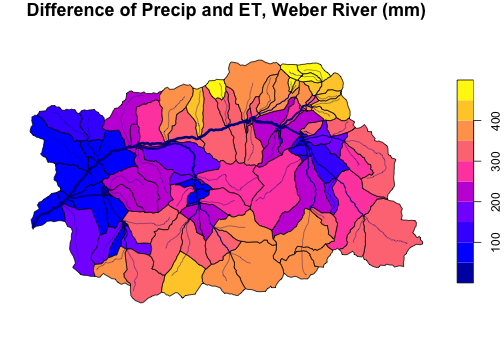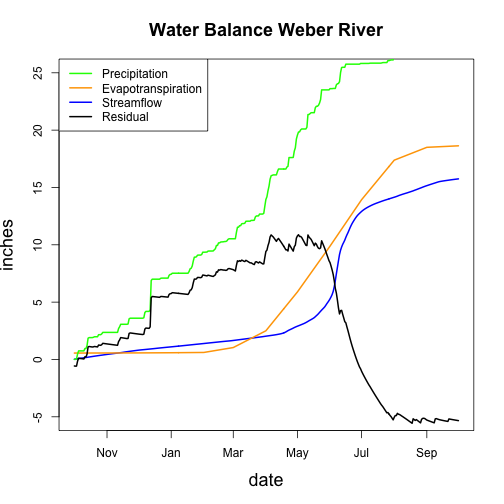Data sourced from web services using R packages shown later.

Application 2: Trends and Plotting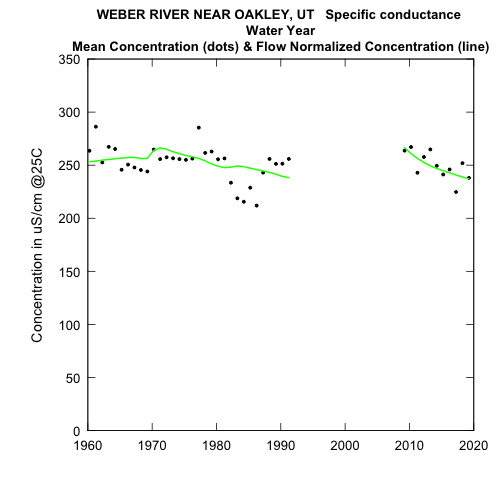Exploration and Graphics for RivEr Trends (EGRET): A package for analysis of long-term changes in water quality and streamflow.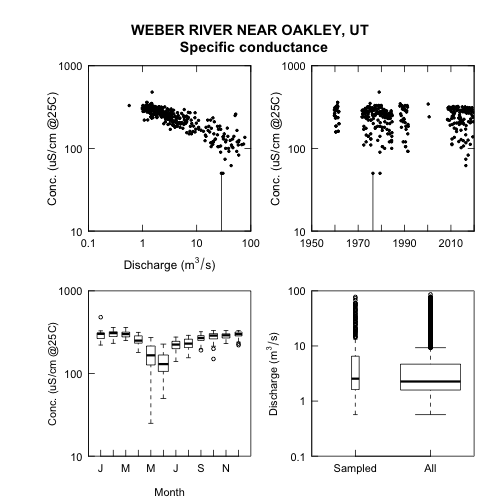Background:

### Spatial Data Access

See a nhdplusTools tutorial here.

``````library(nhdplusTools)
site <- list(featureSource = "nwissite",
featureID = "USGS-10128500")

line <- navigate_nldi(site, "UT", "")
site <- navigate_nldi(site, "UT", "nwissite")

nhdp <- subset_nhdplus(ut\$nhdplus_comid,
"nhdp_subset.gpkg",
``````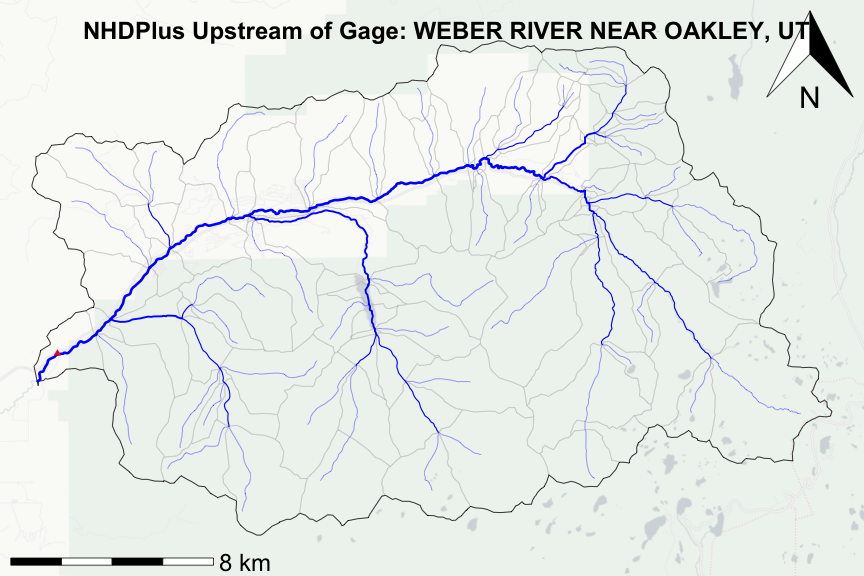### Observations Data Access

Get USGS and EPA water data. See a dataRetrieval tutorial here.

``````library(dataRetrieval)
``````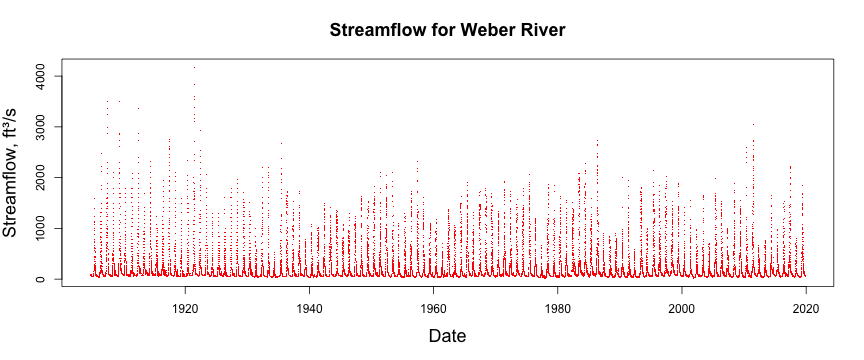Spatial data visualization can be accomplished in several ways. This example uses base R plotting and the `prettymapr` and `rosm` packages. Output is shown on the next slide.

``````prettymap(title = paste("NHDPlus and NLDI data for the", outlet_name),
scale.label.cex = 2, scale.padin = c(0.25, 0.25),
drawarrow = TRUE, arrow.scale = 2,
mai = c(0.5, 0, 0, 0), { # margin for legend
osm.plot(nhd_bbox, type = "cartolight", quiet = TRUE, progress = "none")
plot(gt(nhd_cat), lwd = 0.5, col = NA, border = "grey", add = TRUE)
plot(gt(nhd_basin), lwd = 1, col = NA, border = "black", add = TRUE)
plot(gt(nhd_fline), lwd = streamorder, col = "blue", add = TRUE)
plot(gt(nhd_area), col = "lightblue", border = "lightblue", add = TRUE)
plot(gt(nhd_wbody), col = "lightblue", border = "lightblue",add = TRUE)
plot(gt(wqpsite), col = "red", pch = 46, cex = 1.25, add = TRUE)
plot(gt(nwissite), col = "black", bg = "lightgrey", pch = 24, add = TRUE)})
``````

See the project source code for legend code.
While extremely configurable, base-R plotting can be tedious. `ggplot2` offers a different approach to plotting that some find more convenient.